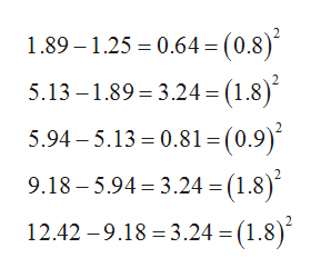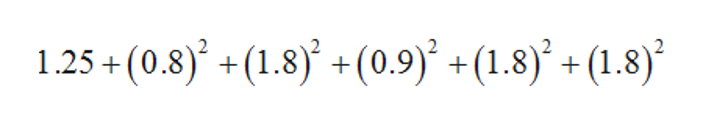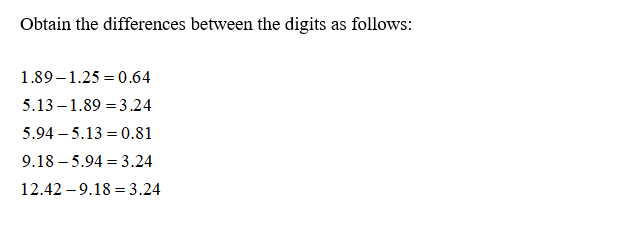# ! =1.89 – 1.25 = 0.64 = (0.8)5.13 – 1.89 = 3.24 = (1.8)´5.94 – 5.13 = 0.81=(0.9)´9.18 – 5.94 = 3.24 = (1.8)´*12.42 –9.18 = 3.24 = (1.8) 1.25 + (0.8)* + (1.8) +(0.9) +(1.8)* + (1.8)*

Question
6 views
1. A pet store offered a baby monkey for sale at \$1.25. The monkey grew, and the next week it was offered at \$1.89.  Not one to monkey around, the shopkeeper subsequently raised the price to \$5.13, then to \$5.94, and next to \$9.18.  Finally, during the sixth week, an organ grinder bought the monkey at \$12.42.  How were the prices figured?
2. The amount of baby monkey is \$1.25; the next week it is offered at \$1.89.
3. Then, raised to \$5.13, then raised to \$5.94, next to \$9.18. Finally, the sixth week the amount at which it was sold is \$12.42.
4. Find the differences between the digits as follows:

The sequence obtained starting from \$1.25 is

As the pattern is not obtained, it can be assumed that there is some unfixed rate which is squared and added to each week price.

Thus, the prices are figured in an uneven manner.help_outlineImage Transcriptionclose! = 1.89 – 1.25 = 0.64 = (0.8) 5.13 – 1.89 = 3.24 = (1.8)´ 5.94 – 5.13 = 0.81=(0.9)´ 9.18 – 5.94 = 3.24 = (1.8)´ * 12.42 –9.18 = 3.24 = (1.8) fullscreenhelp_outlineImage Transcriptionclose1.25 + (0.8)* + (1.8) +(0.9) +(1.8)* + (1.8)* fullscreen
check_circle

Step 1

Since there are multiple questions the first question is being answered. Kindly repost if any particular question has to be answered.

Step 2

1) Given that a pet store offered a bay monkey for sale at \$1.25, again it was offered at \$1.89, the price was raised to \$5.13 and then to \$5.94 ,\$9.18.Finally an organ grinder bought the monkey at \$12.42.

Step 3...

### Want to see the full answer?

See Solution

#### Want to see this answer and more?

Solutions are written by subject experts who are available 24/7. Questions are typically answered within 1 hour.*

See Solution
*Response times may vary by subject and question.
Tagged in